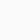# Converting VHDL code snippet to Verilog, issue with signed numbers.Translating VHDL code to Verilog can sometimes be straightforward, but it can also involve dealing with differences in syntax and handling of data types. One common issue when converting VHDL to Verilog is handling signed numbers, as you mentioned. VHDL has built-in support for signed and unsigned data types, while Verilog typically uses two’s complement integers. Here’s how you can handle signed numbers when converting VHDL code to Verilog:

Let’s say you have VHDL code like this, using a signed data type:

vhdl
`signal signed_num : signed(7 downto 0);`

In Verilog, you can represent signed numbers using 2’s complement integers. For an 8-bit signed number, you can use an 8-bit vector and handle it as follows:

verilog
`reg [7:0] signed_num; // Example of assigning a value to signed_num in Verilog always @(posedge clk) begin signed_num <= 8'sb11011011; // This is equivalent to -37 in 2's complement end`

In Verilog, negative numbers are represented using 2’s complement, just like in many hardware description languages. The key is to define your signals and variables as standard vectors, and the interpretation of signed or unsigned depends on how you use them in your design.

Here’s a basic example of converting VHDL code that uses a signed type to Verilog:

VHDL (signed type):

vhdl
`signal input_data : signed(7 downto 0); signal output_data : signed(7 downto 0); output_data <= input_data + 8;`

Equivalent Verilog:

verilog
`reg [7:0] input_data; reg [7:0] output_data; always @(posedge clk) begin output_data <= input_data + 8'b00001000; // Adding 8 to the input_data end`

In Verilog, the operations on `input_data` are performed on an 8-bit vector, which is treated as signed due to the two’s complement representation. Just make sure to handle any sign extensions or bit widths as needed for your specific design.

### Philips TV Model 32PFS6855/12: 3-Minute Operation and Restart### Liquid sensors